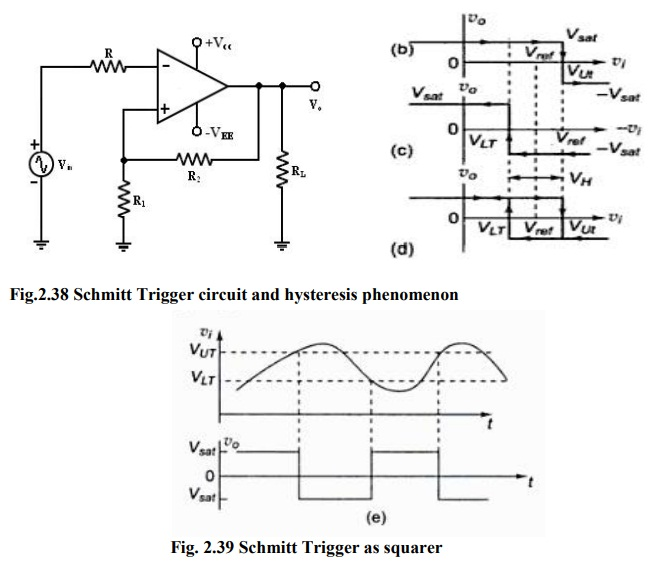Home | | Linear Integrated Circuits | Schmitt Trigger: [Square Circuit]

# Schmitt Trigger: [Square Circuit]This circuit converts an irregular shaped waveform to a square wave or pulse.

Schmitt Trigger: [Square Circuit]

This circuit converts an irregular shaped waveform to a square wave or pulse. The circuit is known as Schmitt Trigger or squaring circuit. The input voltage Vin triggers (changes the state of) the o/p V0 every time it exceeds certain voltage levels called the upper threshold Vut and lower threshold voltage.

These threshold voltages are obtained by using the voltage divider R1– R2, where the voltage across R1 is feedback to the (+) input. The voltage across R1is variable reference threshold voltage that depends on the value of the output voltage. When V0 = +Vsat, the voltage across R1 is called upper threshold voltage Vut.The input voltage Vin must be more positive than Vut in order to cause the output V0 to switch from +Vsat to -Vsat using voltage divider rule,

Voltage at (+) input terminal is VUT=Vref + R2 (Vsat-Vref)/(R1+R2) when V0= +vsat.

When v0= -vsat. Hysteris width VH= VUT - VLT = 2 R2 (Vsat)/ (R1+R2)

When V0 = -Vsat, the voltage across R1 is called lower threshold voltage Vlt .the Vin must be more negative than Vlt in order to cause V0 to switch from –Vsat to +Vsat. for Vin > Vlt , V0 is at –Vsat.

Voltage at (+) terminal is VLT=Vref - R2 (Vsat+Vref)/(R1+R2).

·           If the threshold voltages Vut and Vlt are made larger than the input noise voltages, the positive feedback will eliminate the false o/p transitions.

·           Also the positive feedback, because of its regenerative action, will make V0 switch faster between +Vsat and –Vsat.

·           Resistance Rcomp=R1 || R2 is used to minimize the offset problems.

·           The comparator with positive feedback is said to exhibit hysteresis, a dead band condition. (i.e) when the input of the comparator exceeds Vut its output switches from +Vsat to –Vsat and reverts to its original state, +Vsat when the input goes below VLT. The hysteresis voltage is equal to the difference between Vut and Vlt. Therefore

VH= Vut – Vlt.

·           If Vref=0, Vut= -VLT =2 R2(Vsat)/(R1+R2)Tags : Applications of Operational Amplifier , Linear Integrated Circuits : Applications of Operational Amplifier
Study Material, Lecturing Notes, Assignment, Reference, Wiki description explanation, brief detail
Linear Integrated Circuits : Applications of Operational Amplifier : Schmitt Trigger: [Square Circuit] | Applications of Operational Amplifier